Re-thinking Pythagoras: Is a triangle obtuse?

The Pythagorean Theorem is one of the most remarkable theorems in all of mathematics.  It has a treasure trove of ramifications up its sleeve, any one of which could provide you with invaluable help on the GMAT Quantitative section.  For example, consider this practice problem.

1) Consider the following three triangles

I. a triangle with sides 6-9-10
II.  a triangle with sides 8-14-17
III. a triangle with sides 5-12-14

Which of the following gives a complete set of the triangles that have at least one obtuse angle, that is, an angle greater than 90°?

(A) I
(B) II
(C) III
(D) I & II
(E) II & III

The basic theorem

Everyone knows the basic formula: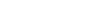To distinguish this formula from the theorem itself (something not often done), I will refer to that as the “Pythagorean formula.”  Many folks don’t realize that the Theorem is something different from this single formula.  Some people realize that, in order for this formula to work, the triangle must be a right triangle, and some people even remember that “c” has to be the hypotenuse of a right triangle.  This leads to the basic statement of the theorem:

If a triangle is a right triangle with sides a < b < c, then the Pythagorean formula is true of the sides.

One important thing to appreciate about Mr. Pythagoras’ famous theorem is that it goes both ways: in logical parlance, it is “biconditional.” In other words

A. If you know the triangle is a right triangle, if you are given that fact, then you can conclude that the Pythagorean formula works for its sides.

and

(B) If you are given the three sides of a triangle, and you know (or can verify) that these three sides satisfy the Pythagorean formula, then that triangle absolutely must be a right triangle.

In other words, if you consider these two qualities (i) being a right triangle, and (ii) sides satisfying the Pythagorean formula, then, those two qualities always come together, and either one necessitates the other.  To use highly dramatic language, God Himself could not create a triangle that has one of those qualities and not the other.

There are common three-number sets know as Pythagorean triplets:  these sets, such as (3, 4, 5), are sets of numbers that satisfy the Pythagorean formula, which necessarily means they would also be the sides of a right triangle.  The reader familiar with the common Pythagorean triplets discussed in that post will find the numbers in the above problem evocative of, but not equal to, these sets of triplets.

Other triangles

Most triangles in the world are not right triangles.  The Pythagorean Theorem applies only to right triangles, but with a little reconfiguring, we can also use it to deduce facts about other triangles.   We know that if there’s an equal sign in the Pythagorean formula, it means the triangle is a right triangle.   What if there’s either a greater-than or a less-than sign instead of equals sign?

Case one: Bigger big side or shorter legs

For this case, we will consider this inequality: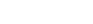This would be true if we started with a right triangle, and then made the hypotenuse bigger while leaving the two legs the same size.   That pushes the two legs apart.This would also be true if we made either one of the smaller, while leave the other two sides the same size.  This pulls the vertex that previously had a right angle closer to the former hypotenuse, which has the effect of pushing the legs apart.In either case, notice that the right angle becomes an obtuse angle.   This allows us to state variation #1 on the Pythagorean Theorem: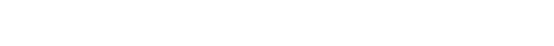Notice: an obtuse triangle is a triangle in which one angle is obtuse.  It is impossible for more than one angle to be obtuse, because then the angles in the triangle would add up to more than 180°.

Case two: Smaller big side or bigger legs

For this case, we will consider this inequality: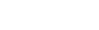This would be true if we started with a right triangle, and then made the hypotenuse smaller while leaving the two legs the same size.   That pulls the two legs toward each other.This would also be true if we made either one of the bigger, while leave the other two sides the same size.  This pushes the vertex that previously had a right angle further from to the former hypotenuse, which has the effect of pulling the legs toward each other.In either case, notice that the right angle becomes an acute angle.   This allows us to state variation #2 on the Pythagorean Theorem: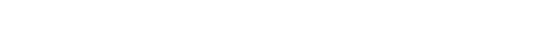Notice: an acute triangle is a triangle in which all three angles are acute.  In any triangle, at least two of the angles must be acute. In an acute triangle, even the largest angle is acute.

Summary

We can combine all this information in one place.  Suppose a triangle has three sides such that a < b < c.  Then:Remembering how to morph the Pythagorean Theorem could help you in a rare GMAT problem such as the one at the top.  More importantly, perhaps this discussion gave you some insight into the interrelationship between math equations and spatial reasoning, and insights along those lines certain could help you in challenging GMAT questions.   Now that you have seen all of this, take another look at the practice problem before reading the solutions below.

Practice problem explanation

1) Remembering the common Pythagorean Triplets is very helpful in this problem.   Triangle I is very close to the (6, 8, 10) right triangle, but we made one of the legs longer.   This makes the sum of the squares of the legs greater than the longest side squared, so Triangle I is acute.   Triangle II is very close to the (8, 15, 17) right triangle, but we made one of the legs shorter.   This makes the sum of the squares of the legs less than the longest side squared, so Triangle II is obtuse.  Triangle III is very close to the (5, 12, 13) right triangle, but we made the longest side bigger.   This makes the sum of the squares of the legs less than the longest side squared, so Triangle III is obtuse.  Answer = E

Author

•Mike served as a GMAT Expert at Magoosh, helping create hundreds of lesson videos and practice questions to help guide GMAT students to success. He was also featured as "member of the month" for over two years at GMAT Club. Mike holds an A.B. in Physics (graduating magna cum laude) and an M.T.S. in Religions of the World, both from Harvard. Beyond standardized testing, Mike has over 20 years of both private and public high school teaching experience specializing in math and physics. In his free time, Mike likes smashing foosballs into orbit, and despite having no obvious cranial deficiency, he insists on rooting for the NY Mets. Learn more about the GMAT through Mike's Youtube video explanations and resources like What is a Good GMAT Score? and the GMAT Diagnostic Test.